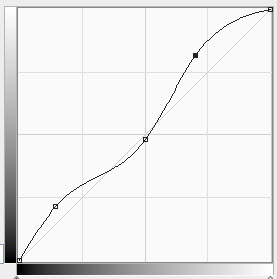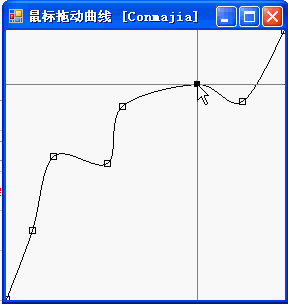# Conmajia

Stop stealing sheep!

## 贝塞尔曲线控件 for .NET (EN)

Conmajia 2012
Updated on Feb. 18, 2018

In Photoshop, there is a very powerful feature Curve Adjust, as shown in figure 1.figure 1 Curve Adjust in Photoshop

You can adjust image parameters by adding, deleting or dragging nodes of the image curve.

Basically, such a curve is simple to implement:

• A curve is presented by a series of points which are called nodes
• Each node is an adjustable handle
• Mouse events handlers for each node

Detailed design procedure is omitted. The result is show in figure 2:figure 2 Animated Example

## Sample Codes

The Node list.

List<Point> points;


Deal with node handlers.

Rectangle getHandle(Point p)
{
Rectangle rect = new Rectangle(
p.X - 3,
p.Y - 3,
6,
6);
return rect;
}


Check for mouse position.

bool isHandle(Point p)
{
foreach (Point pt in points)
{
if (isInside(p, getHandle(pt)))
{
downIndex = points.IndexOf(pt);
downPoint = pt;
current = pt;
return true;
}
}

return false;
}


Draw handlers.

void drawHandle(Graphics g, Point p)
{
if (points.IndexOf(p) == downIndex)
g.FillRectangle(
Brushes.Black,
getHandle(p));
else
g.DrawRectangle(
Pens.Black,
getHandle(p));
}


Draw the curve.

void drawCurve(Graphics g)
{
g.DrawCurve(Pens.Black, points.ToArray());
}


The End. $$\Box$$